# Math Videos

## CA Standards: Algebra II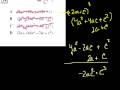Sal from Khan Academy works through polynomial factoring and multiplication problems that involve geometric images. A [...]

## Fractions in lowest termsSal from Khan Academy explains how to simplify complicated fractions to their lowest terms. He explains [...]

## Dividing Decimals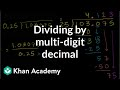Sal from Khan Academy explains how to divide complicated decimal numbers. His explanations help you understand [...]

## Equivalent Fractions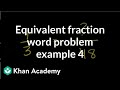Sal from Khan Academy works through complicated fraction word problems to explain the concept of equivalent [...]Sal from Khan Academy explains how to add numbers that contain decimals. His helpful explanation makes [...]

## Changing a Mixed Number to an Improper Fraction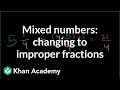Sal from Khan Academy explains how to rewrite mixed numbers as improper fractions. Learn the difference [...]

## Associative Property for Multiplication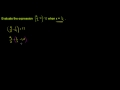Sal from Khan Academy explains the concept behind the associative proper for multiplication. Learn the definition [...]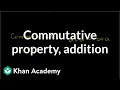Sal from Khan Academy explains the concepts behind the commutative and associative properties of addition. Learn [...]

## Application of Addition of Real NumbersSal of Khan Academy explains how to add numbers that are both positive and negative and [...]

## Dividing Fractions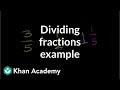Sal from Khan Academy explains how to divide fractions with different denominators. His helpful explanation will [...]